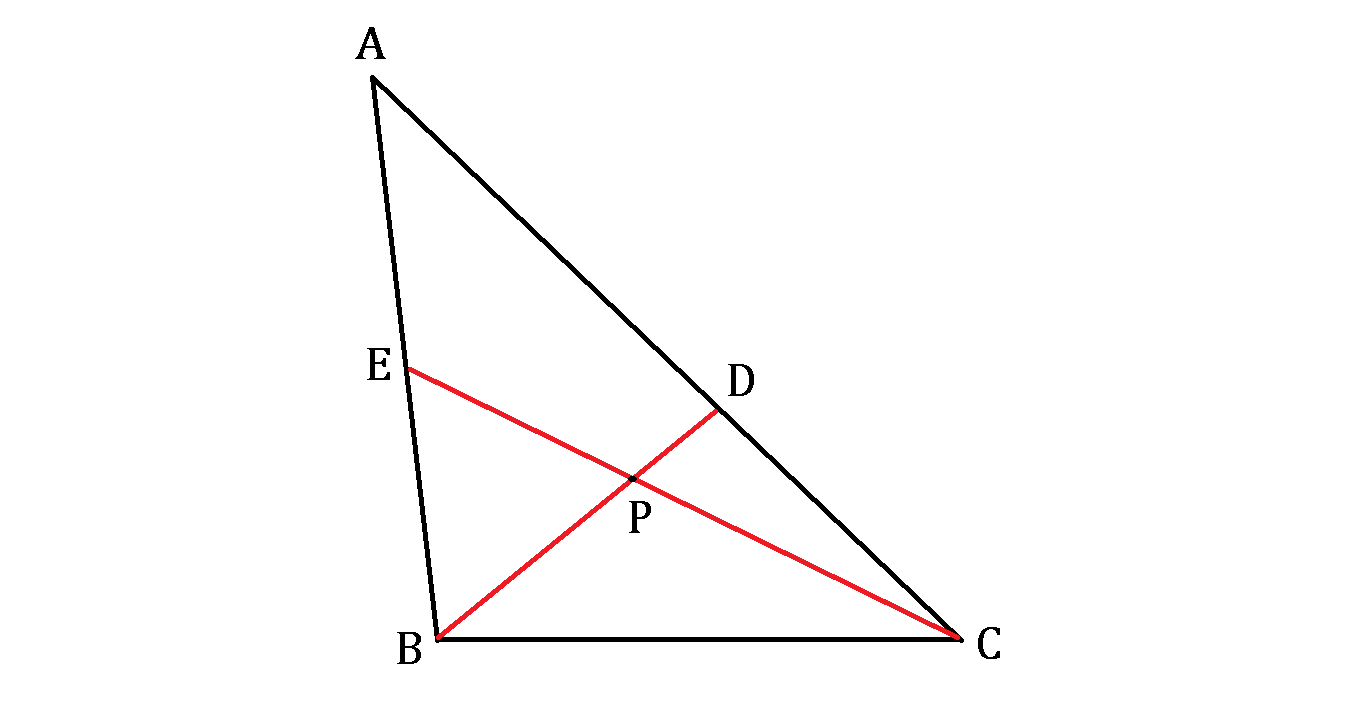# Menelaus' Riddle

Geometry Level 5

Menelaus drew triangle $ABC$ with $BC = 13$ before crossing two red lines $BD = 10$ and $CE = 15,$ both intersecting at point $P$ and reaching the triangle's sides at points $D$ and $E,$ respectively, as shown above.

Menelaus: Mark this, lad. Point $P$ does not only divide all the red segments into integer lengths, but points $D$ and $E$ also divide the triangle's sides into integer lengths.

Pupil: O, so true, master! Any length between any two of those points is always a whole integer!

Menelaus: Then thou shalt tell me. What is the perimeter of triangle $ABC?$×

Problem Loading...

Note Loading...

Set Loading...